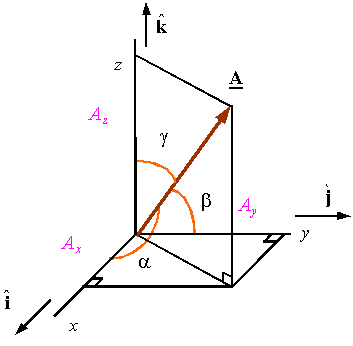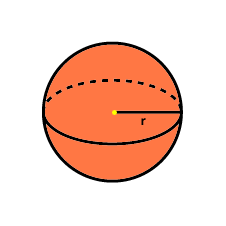Click to Chat

1800-1023-196

+91-120-4616500

CART 0

• 0

MY CART (5)

Use Coupon: CART20 and get 20% off on all online Study Material

ITEM
DETAILS
MRP
DISCOUNT
FINAL PRICE
Total Price: Rs.

There are no items in this cart.
Continue Shopping```3D Geometry

Table of Content

Various Topics Covered in this Chapter Include:​

What do We mean by Direction Cosines of a Line Segment?

What are Direction Ratios?

What is the Condition for Parallel or Perpendicular Lines?

What Exactly is a Sphere?

Related ResourcesThe topic of three dimensional geometry is quite important and a bit complicated as compared to its counterpart two dimensional geometry. One of the ways of describing a 3D object is by approximating or assuming its shape as a mesh of triangles. A triangle is generally defined by three vertices wherein the positions of the vertices are described by the coordinates x, y and z.

The major heads that are included in 3D coordinate geometry are the direction ratios and direction cosines of a line segment.

Various Topics Covered in this Chapter Include:​

Rectangular Co-ordinate System in Space

Direction Cosines of Line

Direction Ratios

Parallel Lines

Projection of Line

Theory of 3D Plane

Theory of 3D Straight Line

Shortest Distance-two Non Intersecting Lines

Theory of Sphere

Solved Problems of 3D Geometry

What do We mean by Direction Cosines of a Line Segment?

The direction cosines are the cosines of the angles between a line and the coordinate axis. If we have a vector (a, b, c) in three dimensional space, then the direction cosines of the vector are defined as

cos α = a/ √(a2 + b2 + c2)

cos β = b/ √(a2 + b2 + c2)

cos γ = c/ √(a2 + b2 + c2)What are Direction Ratios?

If l, m and n are the direction cosines then the direction ratios say a, b and c are given by

l = ± a/√ Σa2

m = ± b/√ Σa2

n = ± c/√ Σa2

Some Key Points:

While the direction cosines of a line segment are always unique, the direction ratios are never unique and in fact they can be infinite in number.

If the direction cosines of a line are l, m and n then they satisfy the relation l2 + m2 + n2 = 1.

If the direction cosines of a line segment AB are (l, m, n) then those of line BA will be (-l, -m, -n).

Angle Between Two Lines

Let us assume that θ is the angle between the two lines say AB and AC whose direction cosines are l1, m1 and n1 and l2, m2 and n2 then

cos θ = l1l2 + m1m2 + n1n2

Also if the direction ratios of two lines a1, b1 and c1 and a2, b2 and c2 then the angle between two lines is given by

cos θ = (a1a2 + b1b2 + c1c2)/ √(  a12 + b12 + c12) . √ (a22 + b22 + c22)

What is the Condition for Parallel or Perpendicular Lines?

When the two lines are perpendicular, the angle between the lines is 90° which gives the condition of perpendicularity as

l1l2 + m1m2 + n1n2 = 0

or this implies a1a2 + b1b2 + c1c2 = 0.

Similarly, when the two lines are parallel, the angle between them i.e. θ = 0.

This gives l1/l2 = m1/m2 = n1/n2

This also gives a1/a2 = b1/b2 = c1/c2

What is the projection of a line segment on a given line?

Suppose we have a line segment joining the points P (x1, y1, z1) and Q(x2, y2, z2), then the projection of this line on another line having direction cosines as l, m, n is AB =  l(x2-x1) + m + m(y2-y1) + n(z2-z1).

What Exactly is a Sphere?

A sphere is basically a circle in three dimensions. Just as a circle, sphere is also perfectly round and is defined as the locus of points that lie at the same distance say ‘r’ from a fixed point in three dimensional space. This ‘r’ is called as the radius of the sphere and this fixed point is called the center of the sphere. The general equation of sphere in 3D is

(x-a)2 + (y-b)2 + (z-c)2 = r2, where (a, b, c) is the center and r is the radius.Illustration: The number of all possible triplets (a1, a2, a3) such that a1 + a2 cos(2x) + a3 sin2x = 0 for all x is zero/infinite/one/ three?

Solution: The given condition is a1 + a2 cos(2x) + a3 sin2x = 0

If we put x = 0 and x = π/2 we get

a1 + a2 = 0 and a1 - a2 + a3 = 0

Hence, a2 = - a1 and a3 = a2 – a1 = -2a1

And so this leads us to the equation of the form

a1 – a1 cos(2x) - 2a1 sin2x = 0 for all x

or a1 (1 – cos(2x) - 2 sin2x) = 0 for all x

which is satisfied for all values of a1

Hence, infinite number of triplets (a1-a1-2a1) are possible.

Illustration: Find the value of k such that (x-4)/1 = (y-2)/1 = (z-k)/2 lies in the plane 2x - 4y + z = 7.

Solution: Given equation of the straight line is

(x-4)/1 = (y-2)/1 = (z-k)/2

Since the line lies in the plane 2x - 4y + z = 7

Hence, the point (4, 2, k) must satisfy the plane which yields

8-8+k = 7

Hence, k = 7.
askIITians is a platform where students get the opportunity of asking any kind of doubts on topics like shortest distance between two lines, equation of plane parallel to xy plane or direction cosines of line along with the solutions of various 3D coordinate geometry problems.

Related Resources

Look into the Previous Year Papers with Solutions to get a hint of the kinds of questions asked in the exam.

You can get the knowledge of Useful Books of Mathematics here.

To read more, Buy study materials of 3D Geometry comprising study notes, revision notes, video lectures, previous year solved questions etc. Also browse for more study materials on Mathematics here.

Watch this Video for more reference

```### Course Features

• 731 Video Lectures
• Revision Notes
• Previous Year Papers
• Mind Map
• Study Planner
• NCERT Solutions
• Discussion Forum
• Test paper with Video Solution